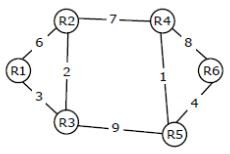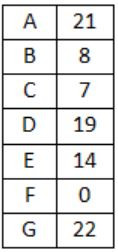## Routing

 Question 1

Consider the following statements about the routing protocols, Routing Information Protocol (RIP) and Open Shortest Path First (OSPF) in an IPv4 network.

• I: RIP uses distance vector routing
• II: RIP packets are sent using UDP
• III: OSPF packets are sent using TCP
IV: OSPF operation is based on link-state routing

Which of the statements above are CORRECT?

 A I and IV only B I, II and III only C I, II and IV only D II, III and IV only
Computer-Networks       Routing       GATE 2017 [Set-2]       Video-Explanation
Question 1 Explanation:
I: RIP uses distance vector routing. “TRUE”
RIP is one of the oldest DVR protocol which employ the hop count as a routing metric.
II: RIP packets are sent using UDP. “TRUE”
RIP uses the UDP as its transport protocol, and is assigned the reserved port no 520.
III: OSPF packets are sent using TCP. “FASLE”
OSPF encapsulates its data directly into IP Packets and does not use either TCP or UDP.
IV: OSPF operation is based on link state routing. “TRUE”
OSPF is a routing protocol which uses link state routing (LSR) and works within a single autonomous system.
 Question 2

Consider the following three statements about link state and distance vector routing protocols, for a large network with 500 network nodes and 4000 links.

[S1] The computational overhead in link state protocols is higher than in distance vector protocols.
[S2] A distance vector protocol (with split horizon) avoids persistent routing loops, but not a link state protocol.
[S3] After a topology change, a link state protocol will converge faster than a distance vector protocol.

Which one of the following is correct about S1, S2, and S3 ?

 A S1, S2, and S3 are all true. B S1, S2, and S3 are all false. C S1 and S2 are true, but S3 is false. D S1 and S3 are true, but S2 is false.
Computer-Networks       Routing       GATE 2014 [Set-1]
Question 2 Explanation:
S1: The computational overhead in link state protocols is higher than in distance vector protocols. Because LSR is based upon global knowledge whereas DVR is based upon Local info.(True)
S2: A distance vector protocol with split horizon avoid persistent routing loops is true, but not a link state protocol is false because link state protocols do not have count to infinity problem.
S3: As Distance vector protocol has count to infinity problem and converges slower. (True)
 Question 3

Which one of the following is TRUE about the interior gateway routing protocols – Routing Information Protocol (RIP) and Open Shortest Path First (OSPF)?

 A RIP uses distance vector routing and OSPF uses link state routing B OSPF uses distance vector routing and RIP uses link state routing C Both RIP and OSPF use link state routing D Both RIP and OSPF use distance vector routing
Computer-Networks       Routing       GATE 2014 [Set-2]
Question 3 Explanation:
RIP Uses Distance Vector Routing and OSPF uses Link State Routing.
 Question 4

Consider a network with five nodes, N1 to N5 as shown below.The network uses a Distance Vector Routing protocol. Once the routes have stabilized, the distance vectors at different nodes are as following.

```N1:(0,1,7,8,4)
N2:(1,0,6,7,3)
N3:(7,6,0,2,6)
N4:(8,7,2,0,4)
N5:(4,3,6,4,0)```

Each distance vector is the distance of the best known path at the instance to nodes, N1 to N5, where the distance to itself is 0. Also, all links are symmetric and the cost is identical in both directions. In each round, all nodes exchange their distance vectors with their respective neighbors. Then all nodes update their distance vectors. In between two rounds, any change in cost of a link will cause the two incident nodes to change only that entry in their distance vectors.

The cost of link N2-N3 reduces to 2(in both directions). After the next round of updates, what will be the new distance vector at node, N3.

 A (3, 2, 0, 2, 5) B (3, 2, 0, 2, 6) C (7, 2, 0, 2, 5) D (7, 2, 0, 2, 6)
Computer-Networks       Routing       GATE 2011
Question 4 Explanation:Question 5

Consider a network with five nodes, N1 to N5 as shown below.The network uses a Distance Vector Routing protocol. Once the routes have stabilized, the distance vectors at different nodes are as following.

```N1:(0,1,7,8,4)
N2:(1,0,6,7,3)
N3:(7,6,0,2,6)
N4:(8,7,2,0,4)
N5:(4,3,6,4,0)```

Each distance vector is the distance of the best known path at the instance to nodes, N1 to N5, where the distance to itself is 0. Also, all links are symmetric and the cost is identical in both directions. In each round, all nodes exchange their distance vectors with their respective neighbors. Then all nodes update their distance vectors. In between two rounds, any change in cost of a link will cause the two incident nodes to change only that entry in their distance vectors.

After the update in the previous question, the link N1-N2 goes down. N2 will reflect this change immediately in its distance vector as cost, infinite. After the NEXT ROUND of update, what will be cost to N1 in the distance vector of N3?

 A 3 B 9 C 10 D ∞
Computer-Networks       Routing       GATE 2011
Question 5 Explanation:
N3 has neighbors N2 and N4
N4 has the distance of 8 to N1
N3 has the distance of 2 to N4
So 2 + 8 = 10
 Question 6

Consider a network with 6 routers R1 to R6 connected with links having weights as shown in the following diagram:All the routers use the distance vector based routing algorithm to update their routing tables. Each router starts with its routing table initialized to contain an entry for each neighbour with the weight of the respective connecting link. After all the routing tables stabilize, how many links in the network will never be used for carrying any data?

 A 4 B 3 C 2 D 1
Computer-Networks       Routing       GATE 2010
Question 6 Explanation:
Link R1- R2 will not be used because its cost is 6 and link R1-R3-R2 costs 5, which is lesser than R1-R2 link.
 Question 7

Consider a network with 6 routers R1 to R6 connected with links having weights as shown in the following diagram:Suppose the weights of all unused links in the previous question are changed to 2 and the distance vector algorithm is used again until all routing tables stabilize. How many links will now remain unused?

 A 0 B 1 C 2 D 4
Computer-Networks       Routing       GATE 2010
Question 7 Explanation:
Now Graph will look likeQuestion 8

Packets of the same session may be routed through different paths in:

 A TCP, but not UDP B TCP and UDP C UDP, but not TCP D Neither TCP nor UDP
Computer-Networks       Routing       GATE 2005
Question 8 Explanation:
Both TCP and UDP uses IP, which is a datagram service.
 Question 9

Two popular routing algorithms are Distance Vector(DV) and Link State (LS) routing. Which of the following are true?

• (S1) Count to infinity is a problem only with DV and not LS routing

• (S2) In LS, the shortest path algorithm is run only at one node

• (S3) In DV, the shortest path algorithm is run only at one node

(S4) DV requires lesser number of network messages than LS
 A S1, S2 and S4 only B S1, S3 and S4 only C S2 and S3 only D S1 and S4 only
Computer-Networks       Routing       GATE 2008-IT
Question 9 Explanation:
→ Count to infinity problem only exist into the DVR.
→ In LSR shortest path is calculated at each every router. (B) is wrong.
→ In DVR also shortest path is calculated at each and every router. (C) is wrong.
→ Since DVR is based upon local knowledge whereas LSR is based upon global knowledge.
 Question 10

For the network given in the figure below, the routing tables of the four nodes A, E, D and G are shown. Suppose that F has estimated its delay to its neighbors, A, E, D and G as 8, 10, 12 and 6 msecs respectively and updates its routing table using distance vector routing technique.ABCDComputer-Networks       Routing       GATE 2007-IT
Question 10 Explanation:
Distance from F to F is 0 which eliminates option (C).
Using distance vector routing protocol, F→D→B yeilds distance as 20 which eliminates option (B) and (D).
 Question 11

A group of 15 routers are interconnected in a centralized complete binary tree with a router at each tree node. Router j communicates with router j by sending a message to the root of the tree. The root then sends the message back down to router j. The mean number of hops per message, assuming all possible router pairs are equally likely is

 A 3 B 4.26 C 4.53 D 5.26
Computer-Networks       Routing       GATE 2007-IT
Question 11 Explanation:
Similarly, average hops when message comes down
= (3×8) + (2×4) + (1×2) + (0×1)/15
= 2.267
So, Total hops = 2×2.267 = 4.53
There are 11 questions to complete.

Register Now